title: “junctions Vignette” author: “Thijs Janzen” date: “2021-05-26” output: rmarkdown::html_vignette vignette: > %\VignetteIndexEntry{junctions} %\VignetteEngine{knitr::rmarkdown} %\VignetteEncoding{UTF-8}

junctions vignette

In this vignette we will take you through the different functions provided in the R package 'junctions', and show how the functions interrelate to each other. In the package junctions we have bundled functions and simulation code to study how ancestry blocks after hybridization decay due to recombination. We assume populations of a constant population size, random mating, and a uniform recombination rate across the chromosome (see the Supplementary material of the paper for scenario's in which we relax some of these assumptions). Furthermore, we assume that no secondary introgression takes place, and we ignore the effect of selection (see the Discussion section of the main paper for a full discussion of the scenario's that are not covered in the model).

An infinite chromosome in a finite population

To simulate the process of junction accumulation over time, we can make use of the function

sim_inf_chrom(pop_size,
initial_heterozygosity,
total_runtime,
morgan,
markers,
seed)

This function has a number of arguments: the population size, the initial heterozygosity, total runtime of the simulation in generations, size of the chromosome in morgan, a flag indicating the number of markers to be used (or -1 if markers are not of interest) and the random seed. The function returns an object containing “avgJunctions”, which is a vector of the average number of junctions in t = [0:total_runtime]. To demonstrate use, we choose some simple parameters:

pop_size <- 100 # population size
h_0 <- 0.5 # initial heterozygosity
maximum_time <- 1000 # run time
c <- 1 # number of recombinations per meiosis

# we first ignore the effect of imposing randomly distributed markers
number_of_markers <- -1

v <- sim_inf_chrom(pop_size = pop_size,
freq_ancestor_1 = h_0,
total_runtime = maximum_time,
morgan = c,
markers = number_of_markers,
seed = 42)

plot(v\$avgJunctions,
type = "l",
xlab = "Generations",
ylab = "Number of Junctions",
main = "Example Infinite Chromosome")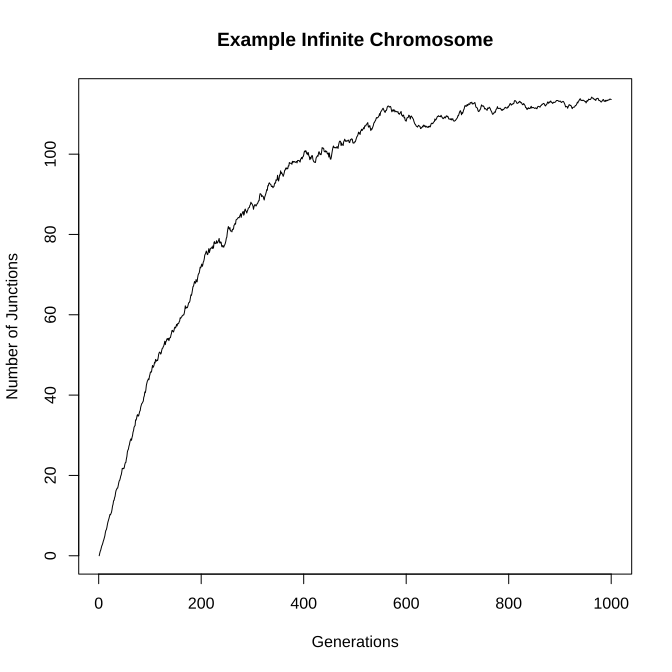Given stochastic junction accumulation, we observe that the number of junctions, although over time increasing, performs a relatively stochastic walk. If we are to compare the accumulation of junctions with our mathematical predictions, we will need to average over a number of replicates.

number_replicates <- 10
v <- c()
for (r in 1:number_replicates) {
v2 <- sim_inf_chrom(pop_size = pop_size,
freq_ancestor_1 = h_0,
total_runtime = maximum_time,
morgan = c,
markers = number_of_markers,
seed = r)
v <- rbind(v, as.numeric(v2\$avgJunctions))
}
v <- colMeans(v) #mean across replicates

Then, we can compare obtained mean estimates with the predicted number of junctions following our mathematical prediction. We do so using the function 'number_of_junctions' (Equation 11 in Janzen et al. 2017)

clarity <- seq(1,
maximum_time,
length.out = 50) # we plot not all points, for clarity
plot(v[clarity] ~ clarity, lwd = 2,
xlab = "Generations",
ylab = "Number of Junctions",
main = "Average behaviour Infinite chromosome", pch = 16)

t <- 0:maximum_time
predicted <- number_of_junctions(N = pop_size, H_0 = h_0, C = c, t = t)
lines(predicted ~ t, col = "blue")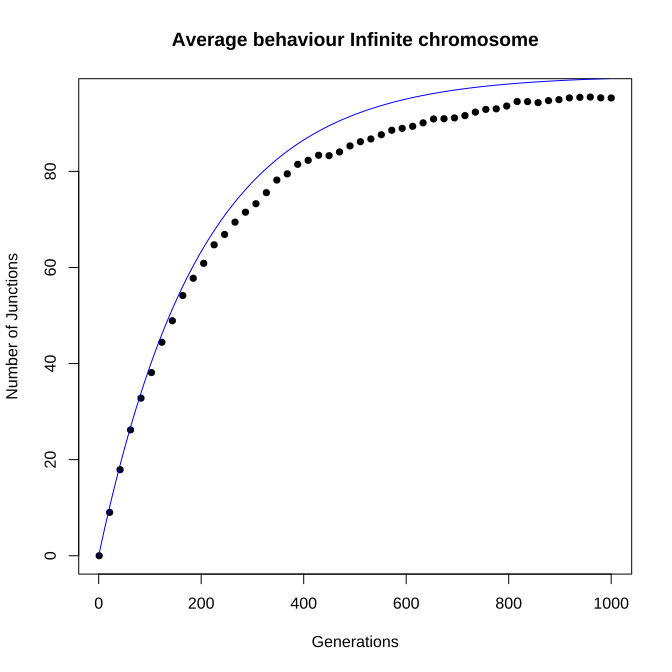We see that the simulations do not exactly match our prediction, but this can be improved by increasing the number of replicates (which we haven't done here, as this would dramatically increase runtime).

Including Random Markers

So far, we have kept the markers flag at -1, this forces the code to ignore the effect of randomly distributed markers. Setting the flag to a positive number R makes the simulation impose R randomly distributed markers upon the chromosome and evaluate the number of junctions based on the genomic content at the locations of these markers. When markers is a positive number, the function “sim_inf_chrom” not only returns a vector “avgJunctions”, but also a vector “detectedJunctions”, which contains the number of junctions detected, given the provided number of markers.

pop_size <- 100 # population size
h_0 <- 0.5 # initial heterozygosity
maximum_time <- 1000 # run time
c <- 1 # number of recombinations per meiosis
number_of_markers <- 1000 # 1000 markers

#single example run
v <- sim_inf_chrom(pop_size = pop_size,
freq_ancestor_1 = h_0,
total_runtime = maximum_time,
morgan = c,
markers = number_of_markers,
seed = 42)
plot(v\$avgJunctions,
type = "l",
xlab = "Generations",
ylab = "Number of Junctions",
main = "Example Infinite Chromosome")

lines(v\$detectedJunctions, col = "blue")
legend("bottomright",
c("Real number", "Number detected"),
lty = 1,
col = c("black", "blue"))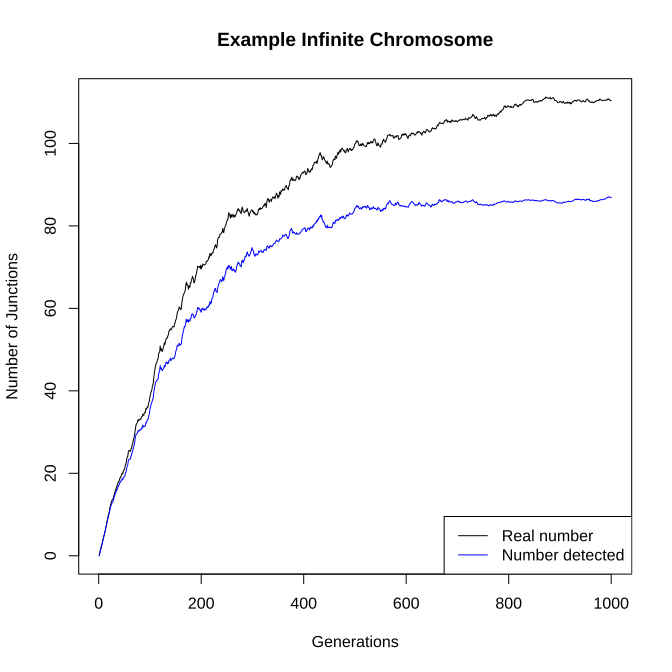We can repeat this analysis again for a number of replicates to acquire mean dynamics:

mean_junctions <- c()
detected_junctions <- c()
for (r in 1:number_replicates) {
v2 <- sim_inf_chrom(pop_size = pop_size,
freq_ancestor_1 =  h_0,
total_runtime = maximum_time,
morgan = c,
markers = number_of_markers,
seed = r + 42)
mean_junctions <- rbind(mean_junctions,
as.numeric(v2\$avgJunctions))
detected_junctions <- rbind(detected_junctions,
as.numeric(v2\$detectedJunctions))
}
mean_junctions <- colMeans(mean_junctions)
detected_junctions <- colMeans(detected_junctions)

We can now plot the true number of junctions, the number detected, and the number predicted on the mathematical analysis. Furthermore, we can substitute K by the maximum number of junctions at the end of the simulation, and use this empirical value of K to predict the number of junctions instead.

#we plot not all points, for clarity
clarity <- seq(1, maximum_time, length.out = 50)
plot(mean_junctions[clarity] ~ clarity,
xlab = "Generations",
ylab = "Number of Junctions",
main = "Average behaviour Infinite chromosome",
pch = 16)

points(detected_junctions[clarity] ~ clarity,
pch = 17,
col = "blue")

t <- 0:maximum_time
predicted <- number_of_junctions(N = pop_size,
H_0 = h_0,
C = c,
t = t)
lines(predicted ~ t, lwd = 2)

# now substitute K with the observed maximum detected.
k <- tail(detected_junctions, 1)
pred <- k - k * (1 - h_0 * c / k) ^ t
lines(pred ~ t, col = "blue", lwd = 2)

legend("bottomright",
c("Real number", "Detected",
"Predicted Real", "Predicted Detected"),
pch = c(16, 17, NA, NA),
lty = c(NA, NA, 1, 1),
col = c("black", "blue",
"black", "blue"),
lwd = 2)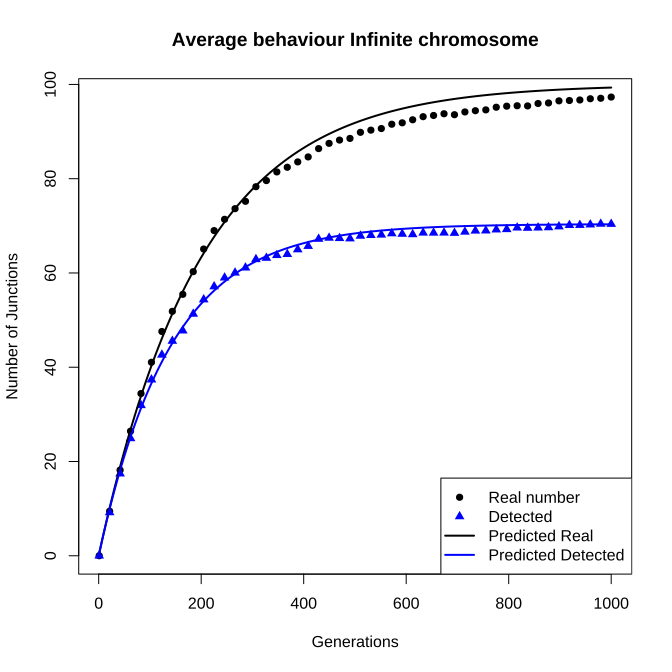A Finite chromosome in a finite population

To simulate a finite chromosome with regularly spaced markers, we use different, more efficient, C code 'under the hood'. For the user to make use of this code, the function 'sim_fin_chrom' can be used:

sim_fin_chrom(pop_size,
initial_heterozygosity,
total_runtime,
morgan,
seed,
R)

Arguments for the function 'sim_fin_chrom' are identical to 'sim_inf_chrom', excluding the markers flag, but including parameter R, indicating the number of genetic markers used. Usage is identical too, and we can obtain a single run as follows:

r <- 100 # chromosome size
n <- 100 # population size
freq_ancestor_1 <- 0.5 # frequency of ancestor 1 at t = 0
c <- 1 # number of recombinations per meiosis
maximum_time <- 1000

#single example run
v <- sim_fin_chrom(pop_size = n,
freq_ancestor_1 = freq_ancestor_1,
total_runtime = maximum_time,
morgan = c,
seed = 42,
R = r)
plot(v\$avgJunctions, type = "l",
xlab = "Generations",
ylab = "Number of Junctions",
main = "Example Finite Chromosome")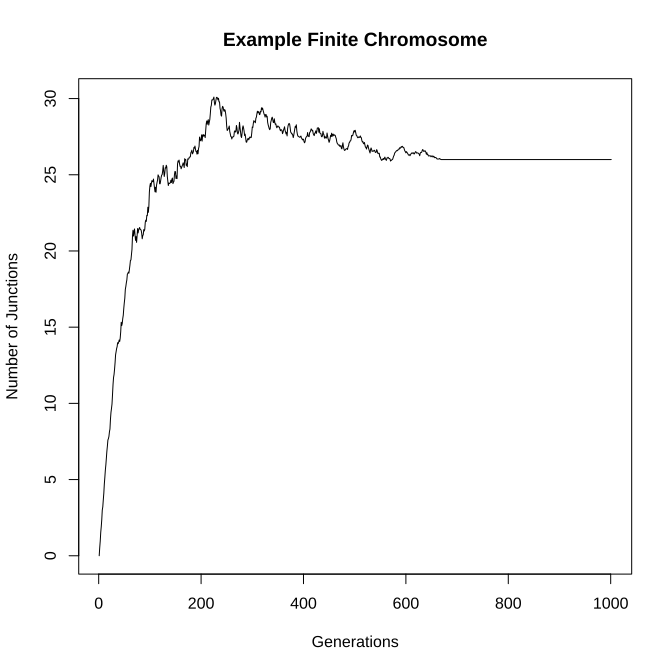To facilitate comparison with empirical data, we might again want to take the mean of a number of replicates:

v <- c()
for (repl in 1:number_replicates) {
v2 <- sim_fin_chrom(pop_size = n,
freq_ancestor_1 = h_0,
total_runtime = maximum_time,
morgan = c,
seed = repl,
R = r)
v <- rbind(v, as.numeric(v2\$avgJunctions))
}
v <- colMeans(v)

Then, using the function 'number_of_junctions', but this time providing the argument R, we can compare our findings with our mathematical predictions again:

clarity <- seq(1, 1000, length.out = 50) #we plot not all points, for clarity
plot(v[clarity] ~ clarity, lwd = 2,
xlab = "Generations",
ylab = "Number of Junctions",
main = "Average behaviour Finite Chromosome",
pch  = 16)

t <- 0:maximum_time
predicted <- number_of_junctions(N = n, R = r,
H_0 = h_0, C = c,
t)
lines(predicted ~ t, col = "blue")
legend("bottomright", c("Simulated", "Predicted"),
pch = c(16, NA),
lty = c(NA, 1),
col = c("black", "blue"))